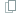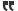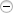# Thread: The easy way to calc probabilities for your deck designs

1. ## The easy way to calc probabilities for your deck designs

When building decks and evaluating how consistently they'll play, many people struggle with how to calculate the probability of:
1. Getting at least one specific card A by the turn they need it to appear, while balancing that probability against having too many of that card when they don't want it to appear.
2. Getting a combo of card A AND card B, either by the same turn or card A on turn X and card B on turn Y.
3. Getting EITHER card A OR card B by a specific turn.
4. Extrapolating from specific cards to general events, the odds of both event A AND event B happening, or of EITHER event A OR event B happening.

1 - How to calculate the probability for any number of the same card on a specific turn

Let's tackle the first bullet, because it's the basis for how to answer all the other bullets.
There is one "best-fit" formula for finding the basic probability inherent in all these types of questions that revolve around card decks. It's called a hypergeometric distribution. Excel has a function for this type of distribution, but it's even easier in many cases to use a free web-based calculator. Here's the best one I've found. It also has decent links to educational/tutorial material on probability theory too.

Start here: http://stattrek.com/Tables/Hypergeometric.aspx

Then plug in the following values:

A - Population size: Your deck size minus 1 (to account for your hero card). For example, if you have a 31-card deck, this value must be 30. If you have a 35-card deck, this value should be 34, etc.

B - Sample size: How many cards you've drawn so far by a given turn. For players with the first turn, this value must be: 6 for turn one, 7 for turn two, 8 for turn three, etc. For players with the second turn, this value must be: 7 for turn one, 8 for turn two, 9 for turn three, etc.

C - Number of successes in the population: How many copies of this card you have in your deck. For example, if you're checking probability for pulling two Sandras by turn five, and you have 4 Sandras in your deck, this value must be 4.

D - Number of successes in sample: How many copies of this card are you testing for? For example, if you're checking probability for pulling two Sandras by turn five, this value must be 2.

You can play with these numbers all you like:

• Change the value of A to test for different deck sizes
• Change the value of C to test for different amounts of each card in your deck. For example, 2 Aeons versus 3 Aeons.

You folks looking for a leg up on building a high-probability deck: there ya go.. this is the golden key that all the tourney MtG players use to build their decks.

2 - How to calculate AND probabililties

Per the second bullet above, it's very easy to calculate the probability of card A AND card B, either on the same turn or different turns.

First, understand that this is different from the probability of having two or three of the *same* card by a given turn. The calculator itself can tell you that by simply using 2 or 3 for the D value.

But what if you want to know the probability for drawing something like "at least one copy of card X on turn 2" AND "at least one copy of card Y on turn 4"? To figure this, you simply multiply the probability for one event against the probability for the other event. The result is your probability for BOTH events occurring.

(Px)(Py) = PxANDy

For example, use the calculator to get the probability for card X on turn 2, then do it again for card Y on turn 4, and then multiply those two probabilities together. The result is the probability that you'll actually land card X on turn 2 AND ALSO land card Y on turn 4.

3 - How to calculate OR probabilities

Per the 3rd bullet above, it's very easy to calculate the probability of card A OR card B, either on the same turn or different turns. In this case, you simply ADD the two probabilities together, then divide by 2.

(Px + Py) / 2 = PxORy

4 - How to calculate probabilities for any combination of AND and OR scenarios

Just throwing this in for good measure. You can use the same basic formulas for AND or OR probabilities to figure the odds of any number of events occurring in any combination of AND or OR clauses.

For example, you want to know the probability of having two Sparks on the board by turn 2 or of having one Sparkwith an Extra Sharpon it by turn 2. Start by breaking this down into separate AND and OR clauses:

Clause A: (1x Sparkon turn 1 AND 1x Sparkon turn 2)
Clause B: (1x Sparkon turn 1 AND 1x Extra Sharpon turn 2)

Now work out Clause A by figuring the odds for at least one Sparkon turn 1, then for at least one Spark on turn 2 (and be sure to reduce the total number of Sparks in the deck to 2 for the second part, because you're assuming you pulled one of them on turn 1). Now multiply those two values together per the rules for an AND probability. Now you have your probability for Clause A.

Work out Clause B in the same manner as for Clause A.

Now use the rules for an OR probability to put Clause A and Clause B together:

(Pa + Pb) / 2 = PaORb

Final note - Beware temptation to make your decks larger than the minimum size allowed by the game rules

Beware the temptation to add just one or two "extra cards" because they're "so good" and they seem to drop the probabilities you care about by only 3% or 4%. Top-tier decks usually run at exactly 30 cards for a reason. Ask any MMO player if equipping gear that provides "only" an extra 3% or 4% critical hit chance is "uber" or in end-game raiding or PvP. Top-level TCG players will also prize every extra percent chance they can muster for the critical turns of the game (which in this game are usually turns 2 and 4).

There's also the issue of compounding this "small" probability drop for any one card into a larger drop for a card combo that you want. If you really want a particular card to play on your turn 2 and another particular card to play on turn 4, then the resulting probability reduction for getting BOTH cards exactly when you want them will be even greater than the probability drop for just one of the cards. For example, what might be a "mere" 4% drop for either one of the cards would compound to something more like a 6.5% drop for the chance of getting off the combo of BOTH cards.Reply With Quote

2. +1 to sticky it.Reply With Quote

3. Cool ^^

The message you have entered is too short. Please lengthen your message to at least 10 characters.Reply With Quote

4. I'm working on a simulator that will shuffle a given deck and give you probabilities of whether you get a certain card in your starting hand, or the first X turns. I'll put a link here when it's done.

In the mean time, you might like to know that, for a 30 card deck (inc. Hero), the chance of getting at least one copy of a given card in your starting hand is:

69% chance for 4 copies
58% chance for 3 copies
43% chance for 2 copies
24% chance for 1 copy

For a 40 card deck (inc. Hero), the chance of getting at least one copy of a given card in your starting hand is:

56% chance for 4 copies
46% chance for 3 copies
33% chance for 2 copies
18% chance for 1 copy

And, finally, for a 40 card deck (inc. Hero), the chance of getting at least one copy of a given card in your hand after drawing 3 additional cards (i.e. 3 turns without help of Bazaar, Majiya, Bad Santa) is:

71% for 4 copies
60% for 3 copies
45% for 2 copies
26% for 1 copy

So, with a 40-card deck, you have roughly the same chance of getting a given card by turn 3 versus having that card in your opening hand in a 30 card deck. But it's still only 70% chance for 4 copies after 3 draws.

I hope the above was useful in some way.Reply With Quote

5. I've finished v0.3 of the deck simulator: Give it a try

Note: You must enter your deck string in the same format that you see when you make your deck with Orca's deckbuilder.

Sorry for hijacking this thread. I will make another if necessary.Reply With Quote

6.Originally Posted by GondorianDotComI've finished v0.3 of the deck simulator: Give it a try

Note: You must enter your deck string in the same format that you see when you make your deck with Orca's deckbuilder.

Sorry for hijacking this thread. I will make another if necessary.
GDC, I can't figure out how to get the deck ID string out of Orca's tool. If you PM me with your google ID (gmail address or whatnot), I'll grant you access to a Google Docs spreadsheet that I spent about 16 hours coding up for Team A1 to use for detailed deck analysis. Maybe you can get some ideas from it for your analysis tool. It's a real pain to create the hypergeometric lookup arrays and the javascript iteration logic for all the various combinations you need. My tool will calculate probabilities for any number of a specific card or from cc bands within the resource curve out to turn 20, and adjust the probabilities depending on whether you're player 1 or player 2.

My tool's format doesn't lend itself to mass cloning/distribution/use or I'd make it public. I'd be only to happy to have someone create a more globally-accessible web-based tool that does similar things.Reply With Quote

7.Originally Posted by shannongGDC, I can't figure out how to get the deck ID string out of Orca's tool. If you PM me with your google ID (gmail address or whatnot), I'll grant you access to a Google Docs spreadsheet that I spent about 16 hours coding up for Team A1 to use for detailed deck analysis. Maybe you can get some ideas from it for your analysis tool. It's a real pain to create the hypergeometric lookup arrays and the javascript iteration logic for all the various combinations you need. My tool will calculate probabilities for any number of a specific card or from cc bands within the resource curve out to turn 20, and adjust the probabilities depending on whether you're player 1 or player 2.

My tool's format doesn't lend itself to mass cloning/distribution/use or I'd make it public. I'd be only to happy to have someone create a more globally-accessible web-based tool that does similar things.
The deck string should be in the address bar when you choose to View/Edit a deck you've built.

I will send you my gmail address.Reply With Quote

8. Calc is the best way to solve long calculation.And i think Probability Calculator is best option for all the Students.If you want to see the Probability Calculator then go to the link.Reply With Quote

9.Originally Posted by ashishbandhuCalc is the best way to solve long calculation.And i think Probability Calculator is best option for all the Students.If you want to see the Probability Calculator then go to the link.
Link is not working......Reply With Quote

10.Originally Posted by PP Star 4everLink is not working......
The link above seem to be prepended with some unnecessary string. Delete those extra characters will allow the link to work.

http://calculator.tutorvista.com/mat...alculator.htmlReply With Quote

####Posting Permissions

• You may not post new threads
• You may not post replies
• You may not post attachments
• You may not edit your posts
•